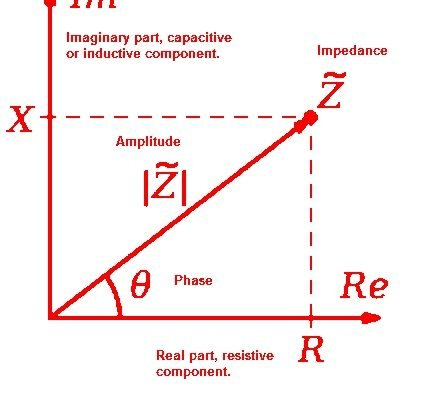## What is impedance

Impedance (Z)  is similar to Resistance (R) . Impedance and Resistance both oppose the current in circuit. Both are almost the same thing , But resistance related to DC Circuit. Resistance oppose the steady electric current in DC circuit. Resistance remains same (constant) at any different frequency range.

Impedance is related with AC circuit. Impedance vary accordting to changing the frequency, this is not constant at different frequency range. Impedance also includes reactance (Inductive and capacitive property of the circuit).

Reactance-  Reactance is the Resistance produced to AC Currents by Inductors and Capacitors only. This is a measure of the type of opposition to AC electricity due to capacitance or inductance.

The impedance is denoted by Z and unit of it is Ohm (Ω).

If the level of ohm is higher then level  impedance is also higher.

Impedance = Resistance + Reactance (Either inductive or Capacitive or both)

In DC circuit, Impedance is effective Resistance of the circuit.

Z= R

In AC circuits, it possesses both magnitude and phase, unlike resistance, which has only magnitude.

In the case of capacitor, When the frequency increased then the resistance (Impedance ) of capacitor decreases. In Inductor this is just opposite, When we increase the frequency range then Impedance increase in inductor.

Impedance

Impedance is defined as combination of resistance and reactance.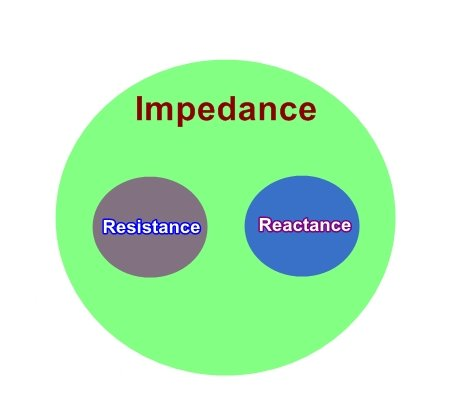As we cannot assume any circuit with DC Current without Resistance , We cannot assume a circuit with AC current without Impedance.

Resistive Power- Energy burns by resistive power to Heat  goes through that system ,

In Reactive Power- the energy goes to Antennas, Speaker,  transmission line, cable etc represents how much energy to be stored and propagates. Not burn to heat ie Impedance.

Resistance     If there is Only Resistor is connected with Load in any circuit then is called Resistor.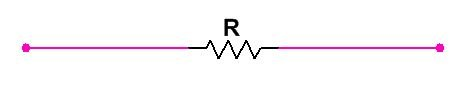• Reactance       If any circuit there is Only inductor or capacitor connected with load. Then the value of v/I is called reactance.

In Reactance There are 2 cases

(1)  If inductor in connected then in this case reactance is called inductive reactance,and its value in scalar form  XL = ѡL,  and in vector form XL=JѡL  Where ѡ=2Πf.  Here If frequency is increased then the value of wL is also increased.

(2) If Capacitor is connected then the Reactance is called Capacitive Reactance and it is denoted by (scalar form) Xc =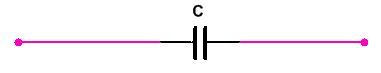In vector form    Where    If frequency (f) is increased then value of Xc is decreased.  ie ω inversly proportional to 2Πf.

♦  Impedance –  If Any circuit consist Resistance -Inductor. Or Resistance - Capacitor, Or Resistance-inductor-Capacitor. Then the value of v/I is called Impedance.

It is denoted by

• If Resistor(R) and Inductor(L) connected –The value of Impedance (scaler form)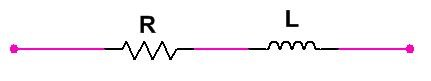In vector form Z= R+jωL

• If Resistor (R) and Capacitor(C) connected -  Then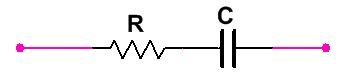Impedance

And In vector form impedance

• If Resistor (R) , Inductor (L) capacitor (C) Connected - Then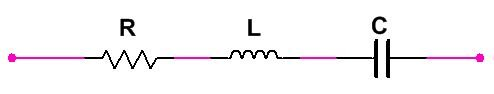Impedance

In vector form

Phasor Diagram of Impedance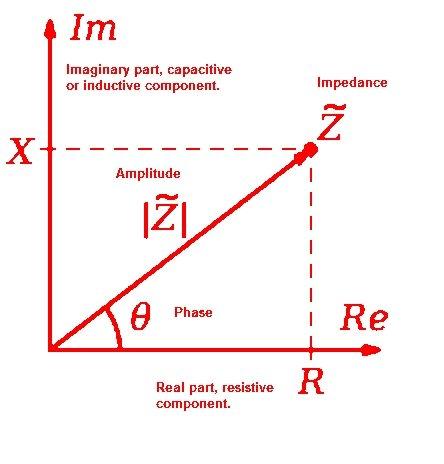Unit

• Impedance - Ω
• Reactance -  Ω
• Resistance - Ω

### About the AuthorHi, My name is Aman bharti, I am interested in making and study of Electronics & circuit diagram. I like to share my knowledge and all ideas with people which i get from My Experiment & from different sources. I try to provide any circuit details deeply with test result as possible as. You can contact me on- Email – [email protected]

## 4 thoughts on “What is Impedance, Impedance vs Resistance”

•Thank you for this post. Its very inspiring.

•very cool post, i actually enjoyed this web site, carry on it

•Much interested have learnt alot from you
Carry on

•It’s in reality a nice and useful piece of info. I’m glad that you just shared this useful info with us. Please stay us informed like this. Thanks for sharing.